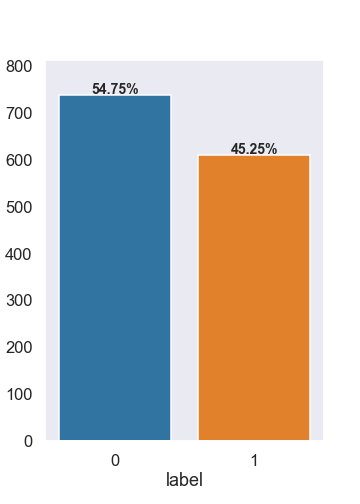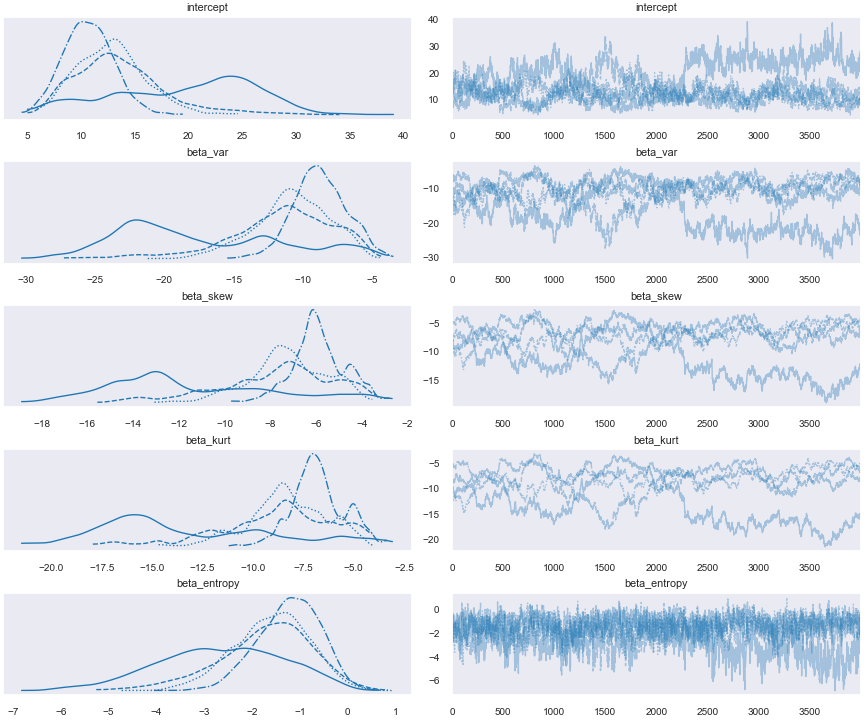top of page
Search

# Bayesian Logistic Regression

1. INTRODUCTION

Authenticating whether any currency note is real or fake is the common problem in financial banks. This problem falls in the category of binary classification problems where we put the observations in one of the two categories. In this article, I have used Bayesian logistic regression to classify currency notes into real or fake classes.

Logistic regression estimates a linear relationship between a set of predictors and a binary target variable. The frequentist approach results in point estimates for the parameters using Maximum Likelihood Estimation(MLE). On the other hand, the Bayesian logistic regression does not find the single best value of the model parameters, but it determines the posterior distribution of the model parameters. The posterior allows us to find more robust estimates (Bayesian credible interval) of each parameter.

2. DATASET

The dataset has been taken from UCI Machine Learning Repository. The data points were extracted from images that were taken from genuine and forged banknote-like specimens. For digitization, an industrial camera usually used for print inspection was used. The final images have 400x400 pixels. Wavelet Transform tool was used to extract features from images.Figure 1: Class distribution and Violin plots

3. EXPLORATORY DATA ANALYSIS

This dataset has 4 predictor variables and 1 response variable. The four predictor variables are variance, skewness, kurtosis and entropy of the wavelet transformed image of the currency notes. The response variable has 2 classes - 0 (fake) and 1 (real). As shown in the left plot of fig 1, the distribution of fake and real notes in the dataset is 54.75% and 45.25% respectively. The right plot of fig 1 shows the distribution of all the predictors with respect to target classes. In this plot we can see that variance and skewness are significantly different for real and fake classes, hence these are the important predictors.

Figure 2 shows the scatter and kde plot between each of the predictors for both the classes. This figure also shows the histogram of each predictors. In these histograms we can see that variance and skewness predictors have relatively less overlap across classes which again emphasises the point that these are important predictors.Figure 2: Pair plot of all predictors

4. MODEL BUILDING METHODOLOGY

To build a Bayesian logistic regression model, we need to put a prior distribution on each parameter. The choice of these priors affects the outcome but with sufficient amount of data generally they converge to the same distribution. I have used non-informative priors i.e. normal distribution with zero mean and very high (1002) variance for all the four predictors and for the intercept. We also need to specify a likelihood in order to draw samples from the posterior. The likelihood is the product of n Bernoulli trials:The figure 3 shows the diagram of the model.Figure 3: Model Digraph plot

I have used Pymc3 to draw samples from the posterior. The code is given in the appendix. PyMC3 numerically approximates the posterior distributions using Markov Chain Monte Carlo (MCMC) simulations. We then use samples from these posteriors to make inferences. I have used Slice MCMC method for sampling. Slice is based on the fact that to sample a random variable, we can sample uniformly from the region under the graph of its density function. I have randomly divided the entire dataset into train and test set (70-30 split) and have used only 70% of the total dataset to train the model.

4.1 Posterior Analysis

The figure 4 shows the posteriors estimates of the parameters.Figure 4: Final equation and posteriors estimates of the parametersFigure 5: Posterior distribution of parameters

The figure 5 shows the posterior distribution of the parameters. The left column shows density plots of the marginal posteriors of each parameter while the right column shows the samples of the Markov chain plotted in sequential order (John Salvatier, 2020). The figure 6 shows the 95% highest posterior density interval (HPD) credible set for all the parameters.4.2 Prediction on hold-out data

The sample posterior predictive() function in pymc3 performs prediction on hold-out data. The fig 7 shows the confusion matrix for the test dataset. The model gives 99.26% accuracy on test dataset.Figure 7: Inference on hold out dataset

5. SUMMARY

The Bayesian regression model performs well on the unseen hold-out dataset and is able to classify the notes into fake and real categories. I have used non-informative priors which basically let the data speak. Using non-informative prior makes the Bayesian models equivalent to the frequentist models but when we have some information about the priors, Bayesian models generally performs better with lesser data points.

The main advantage of Bayesian models is that we get the posterior distribution over parameters which helps us quantify the uncertainty in the parameters using the credible set/variance of the posterior distribution.

REFERENCES

1. John Salvatier, C. F., Thomas V. Wiecki. (2020). Getting started with pymc3.

2. Lohweg, V. (2012). Banknote authentication data set.

3. pymc3 docs. (2020). General api quickstart